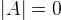# Matrix problem

Given the matrix
A= [a 1 9]
[a -6 3]
[5 -8 a]

find all values of a that makesa can be _ & _

NEED HELP! IM BEYOND STUCK! I have no idea to even approach this problem! Thanks!

Last edited by a moderator:

$$$\left| {\left. {\bf{A}} \right|} \right. = 285 - 48a - 7a^2 = 0$$$